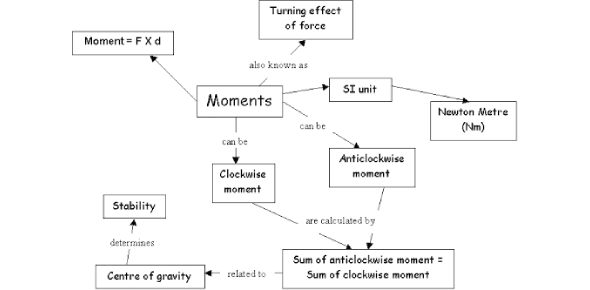# Turning Effect Of Forces Quiz Questions And Answers

10 Questions | Attempts: 8328SettingsWhat do you know about the turning effects of forces? Can you solve these quiz questions and answers based on the turning forces? Try the quiz below and check your knowledge about it. If a force acts on an object, it turns the object around the pivot. The turning force is called the torque. The moment is the product of the force multiplied by the distance from the point an object will turn. Play this quiz and refresh your memory regarding the turning effect of forces.

• 1.
The moment of a force causes an object to
• A.

Move forward

• B.

Move backward

• C.

Stop moving

• D.

• 2.
The turning effect of a force on a door is greater when it is applied at
• A.

A point near the hinges and perpendicular to the door

• B.

A point near the hinges and parallel to the door

• C.

A point far away from the hinges and perpendicular to the door

• D.

A point far away from the hinges and parallel to the door

• 3.
Two equal and oppositely directed forces acting on an object will be in equilibrium
• A.

Only if they act on the same point on the object

• B.

If they are separated by a distance

• C.

When they are balanced by a third force

• D.

when their moments about the same point are equal

• 4.
The diagram shows a light beam pivoted at one end. What is the effort required to lift the load?
• A.

50

• B.

67

• C.

100

• D.

200

• 5.
Jack has a weight of 300 N and sits 2.0 m from the pivot of the see-saw. Jill has a weight of 450 N and sits 1.5 m from the pivot. Who will move down?
• A.

Jack

• B.

Jill

• C.

Both of them

• D.

None of them

• 6.
A nut can be loosen more easily with
• A.

A short spanner because a large turning effect can be produced.

• B.

A short spanner because a larger force can be produced.

• C.

A heavy spanner because a larger force can be produced.

• D.

long spanner because a large turning effect can be produced.

• 7.
The diagram shows a gauge which measures the amount of liquid in a tank. At which position should the pivot be placed so that the pointer moves the greatest distance as the tank is emptied?
• A.

A

• B.

B

• C.

C

• D.

D

• 8.
What is the force F needed to balance the bar shown?
• A.

3

• B.

4

• C.

6

• D.

8

• 9.
A uniform metre rule of weight 16.0 N is pivoted at the 60 cm mark. A 4.0 N weight is suspended from one end. At the instant when the rule is horizontal, what is the value of the resultant turning moment about the pivot?
• A.

Zero

• B.

160 Ncm anticlockwise

• C.

160 Ncm clockwise

• D.

20 N downward

• 10.
Two types of moment of force are
• A.

Forward and backward

• B.

Neutral and charged

• C.

Stable and unstable

• D.

Clockwise and anti-clockwise

## Related TopicsBack to top
×

Wait!
Here's an interesting quiz for you.